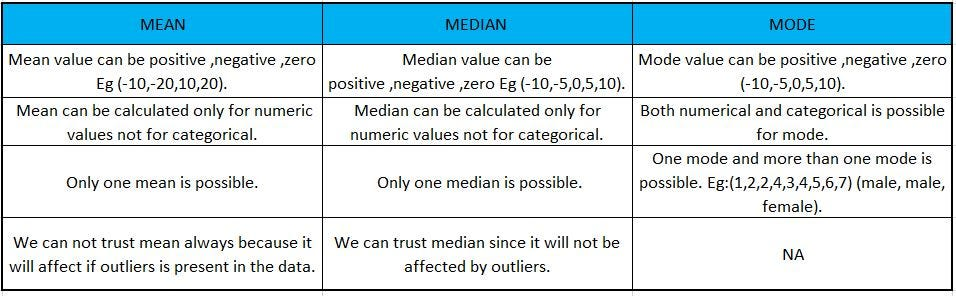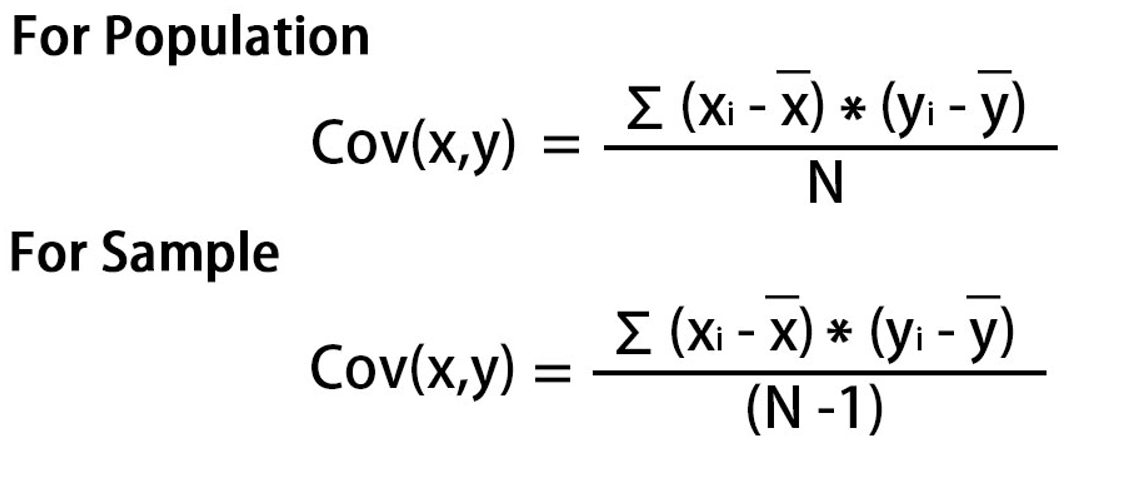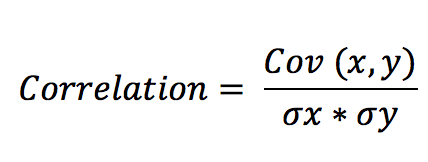# Types of Statistics:

1. Descriptive statistics - Understand the sample
2. Inferential statistics - Understand the population

# Descriptive Statistics:

## 1.Measure of central tendency:

Mean: It is defined as sum of all observation divide by total number of observation.Comparison of Mean , Median ,Mode

## 2. Measures of Spread/Dispersion:

Range: Difference between the Max and Minimum value. (MAX — MIN)

• 25% of the data points lie below Q1 and 75% lie above it.
• 50% of the data points lie below Q2 and 50% lie above it. Q2 is nothing but Median.
• 75% of the data points lie below Q3 and 25% lie above it.
• Mesokurtic — This is the case when the kurtosis is zero, similar to the normal distributions.
• Leptokurtic — This is when the tail of the distribution is heavy (outlier present) and kurtosis is higher than that of the normal distribution.
• Platykurtic — This is when the tail of the distribution is light( no outlier) and kurtosis is lesser than that of the normal distribution.COVARIANCE -TOGETHER SPREAD OF X AND YCORRELATION: COV(x)/SIGMA (x) IS ZSCALED FORMULA SO IT IS DOING SCALING

# INFERENTIAL STATISTICS:

Inferential statistics main aim is to take sample data from population do some statistical test and come to conclusion of the specific data.

1. Starts with data
2. Arriving the insights y=f(x) and finding hypothesis.

--

--## Vignesh S

Data scientist Aspirant passionate in learning new technologies and sharing my thoughts to others .